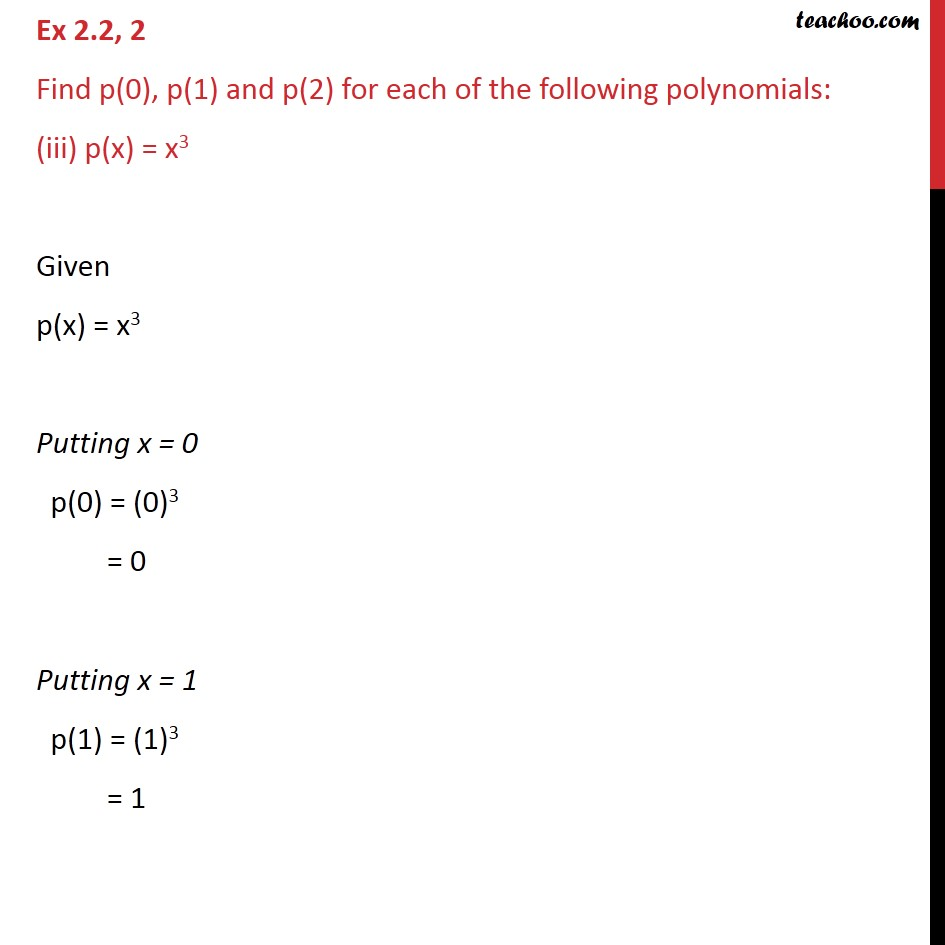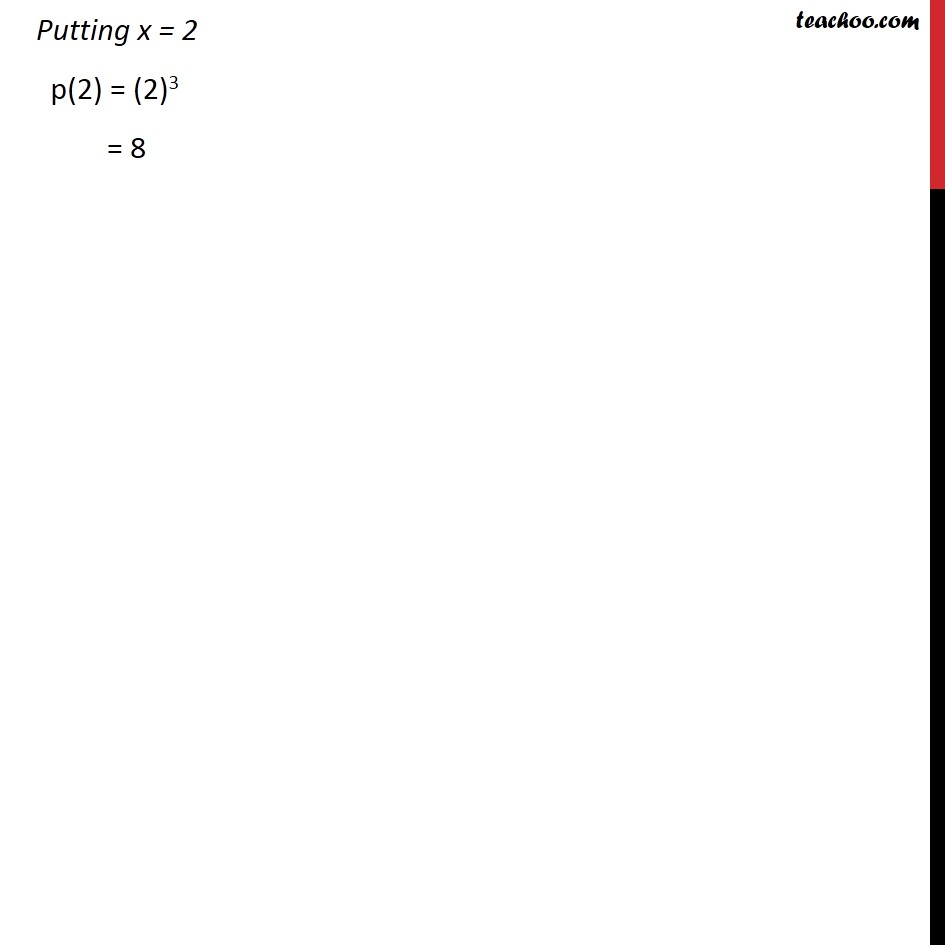Ex 2.2

Chapter 2 Class 9 Polynomials
Serial order wiseLearn in your speed, with individual attention - Teachoo Maths 1-on-1 Class

### Transcript

Ex 2.2, 2 Find p(0), p(1) and p(2) for each of the following polynomials: (iii) p(x) = x3 Given p(x) = x3 Putting x = 0 p(0) = (0)3 = 0 Putting x = 1 p(1) = (1)3 = 1 Putting x = 2 p(2) = (2)3 = 8